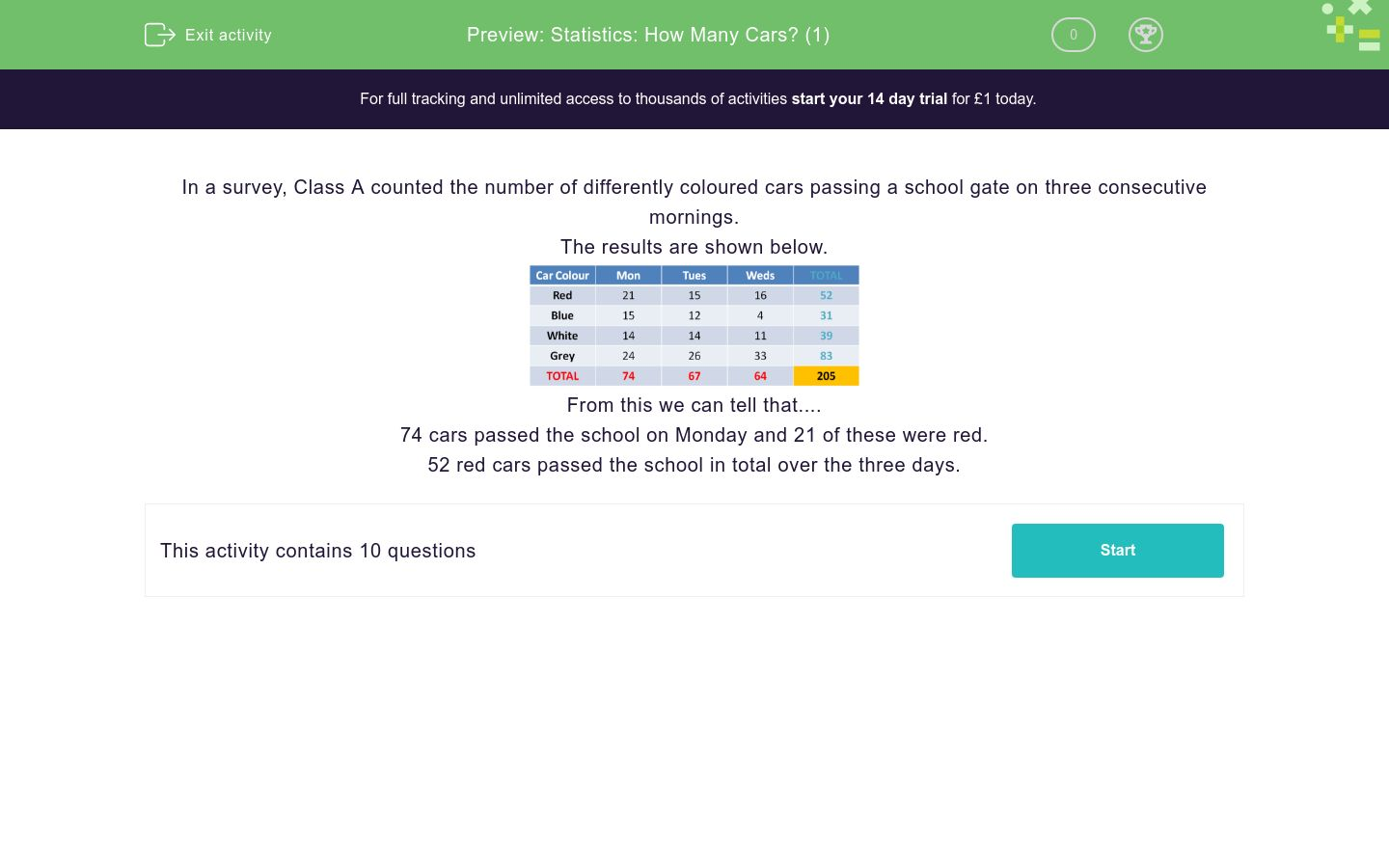# Statistics: How Many Cars? (1)

In this worksheet, students answer questions using the results of a survey shown in a data table.Key stage:  KS 2

Curriculum topic:   Statistics

Curriculum subtopic:   Compare Data Using Charts and Graphs

Difficulty level:### QUESTION 1 of 10

In a survey, Class A counted the number of differently coloured cars passing a school gate on three consecutive mornings.

The results are shown below.From this we can tell that....

74 cars passed the school on Monday and 21 of these were red.

52 red cars passed the school in total over the three days.

Use the table of results to answer the question.

How many blue cars passed on Tuesday?Use the table of results to answer the question.

How many white cars passed on Tuesday?Use the table of results to answer the question.

How many grey cars passed on Monday?Use the table of results to answer the question.

How many white cars passed in total over the three days?Use the table of results to answer the question.

How many red cars passed in total over the three days?Use the table of results to answer the question.

How many grey cars passed in total over Monday and Tuesday?Use the table of results to answer the question.

How many cars passed in total on Wednesday?Use the table of results to answer the question.

How many cars passed in total on Monday?Use the table of results to answer the question.

On which day did most cars pass?Use the table of results to answer the question.

How many cars passed in total over the three days?• Question 1

Use the table of results to answer the question.

How many blue cars passed on Tuesday?12
EDDIE SAYS
Look at the Tuesday column on the chart and find the blue car row. 12 blue cars passed that day.
• Question 2

Use the table of results to answer the question.

How many white cars passed on Tuesday?14
EDDIE SAYS
Locate the Tuesday column and find the row for white cars. 14 white cars passed that day. Well done for carefully reading the question and the table!
• Question 3

Use the table of results to answer the question.

How many grey cars passed on Monday?24
EDDIE SAYS
Find the Monday column and then locate the row for grey cars. 24 cars passed that day.
• Question 4

Use the table of results to answer the question.

How many white cars passed in total over the three days?39
EDDIE SAYS
Locate the Total column and find the number for the white cars - 39. Well done!
• Question 5

Use the table of results to answer the question.

How many red cars passed in total over the three days?52
EDDIE SAYS
Locate the Total column and find the row for red cars - 52.
• Question 6

Use the table of results to answer the question.

How many grey cars passed in total over Monday and Tuesday?50
EDDIE SAYS
Look at the row for grey cars and locate the Monday and Tuesday numbers. Add them together to get your answer: 24 + 26 = 50.
• Question 7

Use the table of results to answer the question.

How many cars passed in total on Wednesday?64
EDDIE SAYS
If you look at the last row of the table, the red numbers are the total numbers for the days. The total number of cars that passed on Wednesday was 64.
• Question 8

Use the table of results to answer the question.

How many cars passed in total on Monday?74
EDDIE SAYS
Look at the red total number in the Monday column - 74.
• Question 9

Use the table of results to answer the question.

On which day did most cars pass?Monday
EDDIE SAYS
Look at the row with the red total numbers and find that Monday most cars passed.
• Question 10

Use the table of results to answer the question.

How many cars passed in total over the three days?205
EDDIE SAYS
Find the total for cars that passed over the three days in the yellow box - 205. Well done for reading the chart carefully!
---- OR ----

Sign up for a £1 trial so you can track and measure your child's progress on this activity.

### What is EdPlace?

We're your National Curriculum aligned online education content provider helping each child succeed in English, maths and science from year 1 to GCSE. With an EdPlace account you’ll be able to track and measure progress, helping each child achieve their best. We build confidence and attainment by personalising each child’s learning at a level that suits them.

Get started